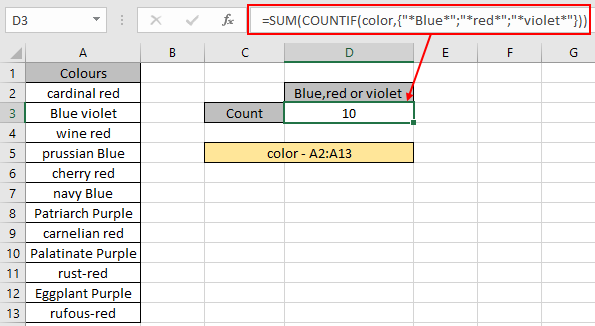# Count cells that have either of a, b or c

In this article, we will learn about how to Count cells that equals either A ,B or C value using COUNTIF & SUM function in Excel.

In simple words, Consider a scenario in which we are required to count the data based on specific criteria in an array. counting cells that have either A ,B or C value using the wildcards to catch cell value with COUNTIF & SUM function in excel explained here with an example. Use Wildcards characters for catching strings and perform functions on it.

The COUNTIF function of excel just counts the number of cells with a specific condition in a given range.

The SUM function adds up all the input numbers and returns the sum.

Use the formula:

= SUM ( COUNTIF ( range , { "value1" ; "*value2*" ; "*value3*" } ) )

Let’s understand this function using it as an example.

Example:There There are different shades of colours in range ( A2:A13 ). We need to find the colours which have either Blue, red or violet.

Use the formula:

= SUM ( COUNTIF ( color , { "*Blue*" ; "*red*" ; "*violet*" } ) )

Color : named range for the array A2:A13
* : Asterisk ( * ) wildcard used to extract value wherever value is found.
The formula drops down to
= SUM ( { 3 ; 6 ; 1 } )
3 Blue, 6 red and 1 violet colorThere are 10 colors which have either Blue, red or violet.

As you can see the COUNTIF formula returns the count of cells having either of A, B or C value. Here are some observations about the formula.

Notes:

1. Wildcards help with extracting value from the substrings.
2. The COUNTIF function supports logical operators like <, >, <>, = but these are used using double quote sign ( " ) .
3. Condition argument should use quote sign (") for texts.

Hope you understood how to Count cells which match either of the three values A , B or C in Excel. Explore more articles on Excel Count functions here. Please feel free to state your query or feedback for the above article.

Related Articles

COUNTIFS with Dynamic Criteria Range

COUNTIFS Two Criteria Match

COUNTIFS With OR For Multiple Criteria

The COUNTIFS Function in Excel

How to Use Countif in VBA in Microsoft Excel

How to use wildcards in excel

Popular Articles

50 Excel Shortcut to Increase Your Productivity

Edit a dropdown list

If with conditional formatting

If with wildcards

Vlookup by date

Terms and Conditions of use

The applications/code on this site are distributed as is and without warranties or liability. In no event shall the owner of the copyrights, or the authors of the applications/code be liable for any loss of profit, any problems or any damage resulting from the use or evaluation of the applications/code.